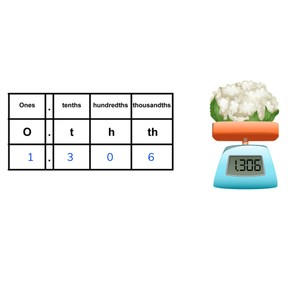Place value- decimal numbers with 3 decimal places

# Place value- decimal numbers with 3 decimal places

Place value- decimal numbers with 3 decimal places8,000 schools use Gynzy92,000 teachers use Gynzy1,600,000 students use Gynzy

## General

Students learn the order of numbers in a decimal number with 3 decimal places. They learn how to place these numbers in a decimal place value chart and to determine the value of digits in a decimal number.

-5.NBT.A.3

## Relevance

It is important to know the parts of a decimal number so students will be able to calculate using these numbers in the future.

## Introduction

Start with a quiz about decimal numbers. Repeat the term "decimal places" to make sure there is understanding and repeat with the students how to say the decimal numbers (and the decimal places) out loud.

## Development

Review with students what tenths and hundredths are. Then explain what thousandths are. You write a thousandth as 0.001, and it fits 1,000 times into 1.000. Check that students understand this by asking questions. How do you say 0.009? Show the different parts of a decimal number with 3 decimal places using the decimal place value chart (tens, ones, tenths, hundredths, and thousandths). Next show how a number can be divided into its place value portions on a number line. The number 14.253 can be split into 10.000+4.000+0.200+0.050 and + 0.003. Discuss the value of the digits in the decimal number: the 1 represents 1 tens and has a value of 10.000. The 4 represents 4 ones and has a value of 4.000. The 2 represents 2 tenths and has a value of 0.200. The 5 represents 5 hundredths and has a value of 0.050. The 3 represents 3 thousandths and has a value of 0.003. Practice setting decimal numbers into the decimal place value chart, reading decimal numbers and filling in the missing decimal number from the steps on the number line with the students.

Use the following questions to check for understanding of decimal numbers with 3 decimal places:
- How many thousandths does the number 4.982?
- Is the number 3 a tenth, hundredth, or thousandth in the number 1.136?
- What is 3.000 + 0.500 + 0.090 + 0.001?

## Guided practice

Students do an exercise with tens, ones, tenths, hundredths, and thousandths. Ask what the total is of these numbers. Next students must complete a decimal place value chart. They then must determine the missing decimal number.

## Closing

Discuss with students why it is important that they know the parts and place value of digits in a decimal number. When you know how to do this, you are able to calculate using decimal numbers. Finish with a quiz on decimal numbers. This time students must determine value of decimals within a number.

## Teaching tips

Make sure that students are comfortable with determining place value of digits in decimal numbers before they begin calculations using decimal numbers.

### The online teaching platform for interactive whiteboards and displays in schools

• Save time building lessons

• Manage the classroom more efficiently

• Increase student engagement